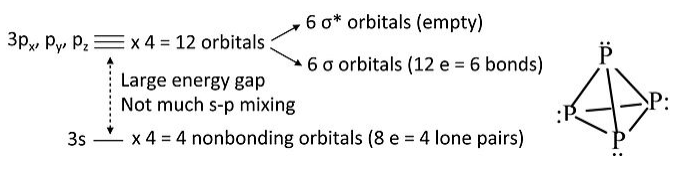# 2.9: Building up the MOs of More Complex Molecules- NH₃, P₄

## MO diagram for NH3

We can now attempt the MO diagram for NH3, building on the result we obtained with triangular H3+.Notes on the MO diagram for ammonia:

• Viewed end-on, a p-orbital or an spx hybrid orbital looks just like an s-orbital. Hence we can use the solutions we developed with s-orbitals (for H3+) to set up the σ bonding and antibonding combinations of nitrogen sp3 orbitals with the H 1s orbitals.
• We now construct the sp3 hybrid orbitals of the nitrogen atom and orient them so that one is “up” and the other three form the triangular base of the tetrahedron. The latter three, by analogy to the H3+ ion, transform as one totally symmetric orbital (“a1”) and an e-symmetry pair. The hybrid orbital at the top of the tetrahedron also has a1 symmetry.
• The three hydrogen 1s orbitals also make one a1 and one (doubly degenerate) e combination. We make bonding and antibonding combinations with the nitrogen orbitals of the same symmetry. The remaining a1 orbital on N is non-bonding. The dotted lines show the correlation between the basis orbitals of a1 and e symmetry and the molecular orbitals
• The result in the 8-electron NH3 molecule is three N-H bonds and one lone pair localized on N, the same as the valence bond picture (but much more work!).

## P4 molecule and P42+ ion:

By analogy to NH3 we can construct the MO picture for one vertex of the P4 tetrahedron, and then multiply the result by 4 to get the bonding picture for the molecule. An important difference is that there is relatively little s-p hybridization in P4, so the lone pair orbitals have more s-character and are lower in energy than the bonding orbitals, which are primarily pσ.Take away 2 electrons to make P42+

Highest occupied MO is a bonding orbital → break one bond, 5 bonds left

Square form relieves ring strain, (60° → 90°)